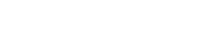# Logic Apps (Standard) Data Mapper: Conversion functions

Posted: July 25, 2023  |  Categories: Azure Azure Integration Services Logic Apps

## Overview

Conversion functions are used to convert values to specific times, such as conversions to DateTime, to integer, to a number, or to string. If you come from the BizTalk Server background or are migrating BizTalk Server projects, they are the equivalent of Conversion Functoids inside BizTalk Mapper Editor. However, the BizTalk Server does not have these conversion types.

## Available Functions

The Conversion functoids are:

• To DateTime: Returns the specified value as a DateTime value.
• To integer: Returns the specified value as an integer.
• To number: Returns the specified value as a number.
• To string: Returns the specified value as a string.

## To DateTime

This function states that it returns the specified value as a DateTime value.

Behind the scenes, this function is translated to a Type casting rule in XQuery: date()

• date(string?)

Rules:

• Castable values are restricted by the target types implementation restrictions. For example, you cannot cast a date string with a negative year to xs:date. Such casts will result in the empty sequence if the value is provided at run time (instead of raising a run-time error).

## To integer

This function states that it returns the specified value as an integer.

Behind the scenes, this function is translated to the following XPath function: number() idiv 1

• `number`(`\$arg as xs:anyAtomicType?`)` as xs:double` idiv 1

Note: idiv is an integer division operator. XPath supports two division operators named `div` and `idiv`. Each of these operators accepts two operands of any numeric type. `\$arg1 idiv \$arg2` is equivalent to `(\$arg1 div \$arg2) cast as xs:integer?` except for error cases.

Rules:

• If `\$arg` is the empty sequence, or if `\$arg` cannot be converted to an `xs:double`, the `xs:double` value `NaN` is returned.
• Otherwise, `\$arg` is converted to an `xs:double` following the rules of casting to xs:double. If the conversion to `xs:double` fails, the `xs:double` value `NaN` is returned.

## To number

This function states that it returns the specified value as a number.

Behind the scenes, this function is translated to the following XPath function:: number()

• `number`(`\$arg as xs:anyAtomicType?`)` as xs:double`

Rules:

• If `\$arg` is the empty sequence, or if `\$arg` cannot be converted to an `xs:double`, the `xs:double` value `NaN` is returned.
• Otherwise, `\$arg` is converted to an `xs:double` following the rules of casting to xs:double. If the conversion to `xs:double` fails, the `xs:double` value `NaN` is returned.

## To string

This function states that it returns the specified value as a string.

Behind the scenes, this function is translated to a Type casting rule in XQuery: string()

• `string string(object?)`

Rules:

• A node-set is converted to a string by returning the string-value of the node in the node-set that is first in document order. If the node-set is empty, an empty string is returned.
• A number is converted to a string as follows
• NaN is converted to the string `NaN`
• positive zero is converted to the string `0`
• negative zero is converted to the string `0`
• positive infinity is converted to the string `Infinity`
• negative infinity is converted to the string `-Infinity`
• if the number is an integer, the number is represented in decimal form as a Number with no decimal point and no leading zeros, preceded by a minus sign (`-`) if the number is negative
• otherwise, the number is represented in decimal form as a Number including a decimal point with at least one digit before the decimal point and at least one digit after the decimal point, preceded by a minus sign (`-`) if the number is negative; there must be no leading zeros before the decimal point apart possibly from the one required digit immediately before the decimal point; beyond the one required digit after the decimal point there must be as many, but only as many, more digits as are needed to uniquely distinguish the number from all other IEEE 754 numeric values.
• The boolean false value is converted to the string `false`. The boolean true value is converted to the string `true`.
• An object of a type other than the four basic types is converted to a string in a way that is dependent on that type.

Hope you find this helpful! So, if you liked the content or found it useful and want to help me write more, you can buy (or help buy) my son a Star Wars Lego!##### Author: Sandro Pereira

Sandro Pereira lives in Portugal and works as a consultant at DevScope. In the past years, he has been working on implementing Integration scenarios both on-premises and cloud for various clients, each with different scenarios from a technical point of view, size, and criticality, using Microsoft Azure, Microsoft BizTalk Server and different technologies like AS2, EDI, RosettaNet, SAP, TIBCO etc. He is a regular blogger, international speaker, and technical reviewer of several BizTalk books all focused on Integration. He is also the author of the book “BizTalk Mapping Patterns & Best Practices”. He has been awarded MVP since 2011 for his contributions to the integration community.Over 650+ customers across
30+ countries depend on BizTalk360